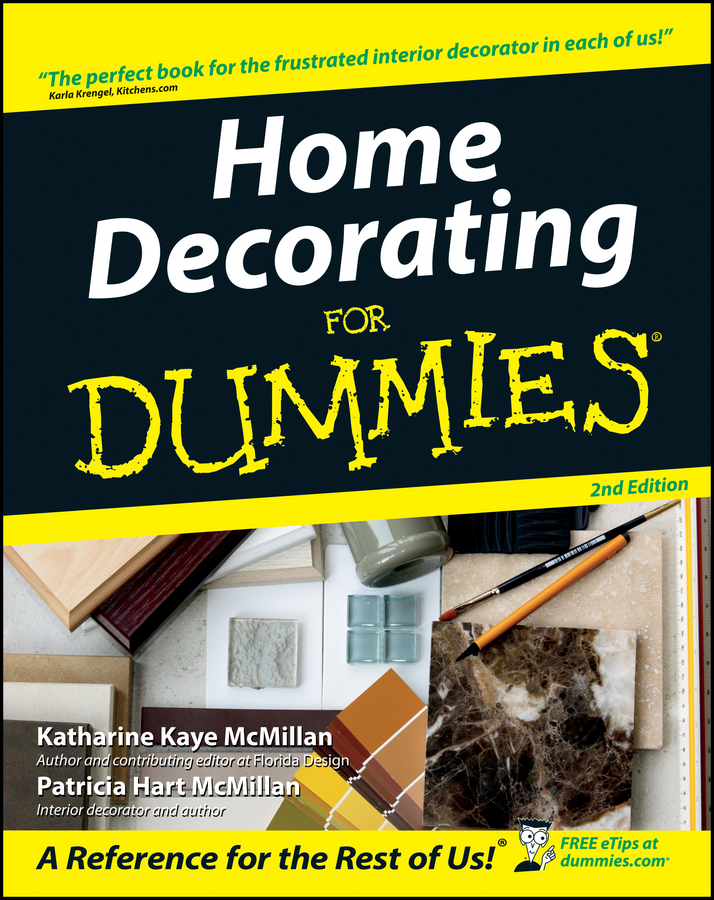##### Home Decorating For DummiesDoes your home improvement project involve a new vinyl floor? To figure out how many vinyl floor tiles, or sheet vinyl, you need, follow these simple formulas:

## Vinyl floor tile calculator

To figure out how many vinyl floor tiles you need to buy, calculate the floor area you want to cover and divide that number by the size of one tile:

Floor Area:

[Length of Floor] × [Width of Floor] = Floor Area

Tiles to Order:

• For 9" Tiles: Floor Area ÷ 0.5625 = Number of 9" Tiles Needed

• For 12" Tiles: Floor Area = Number of 12" Tiles Needed

## Sheet vinyl flooring calculator

Determine how much sheet vinyl to purchase by calculating the square footage of floor you plan to cover and dividing that number by 9 to get the number of square yards of flooring you need.

Floor Area:

[Length of Floor (ft.)] × [Width of Floor (ft.)] = Floor Area (sq. ft.)

Sheet Vinyl to Order:

[Floor Area] ÷ 9 = Number of Sq. Yards of Floor Covering Needed